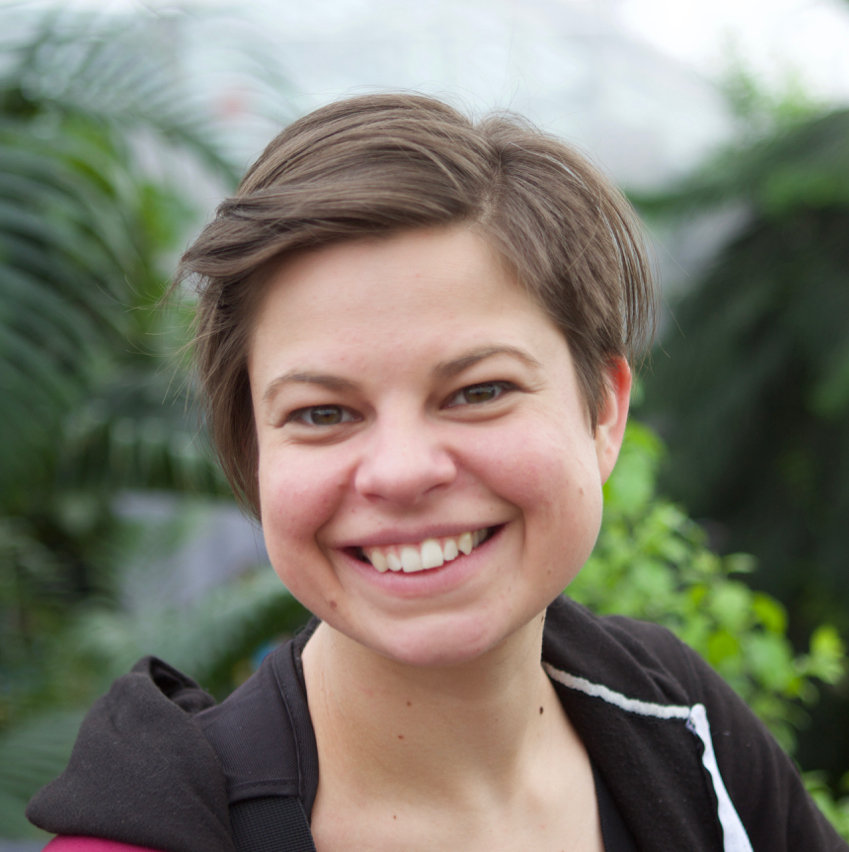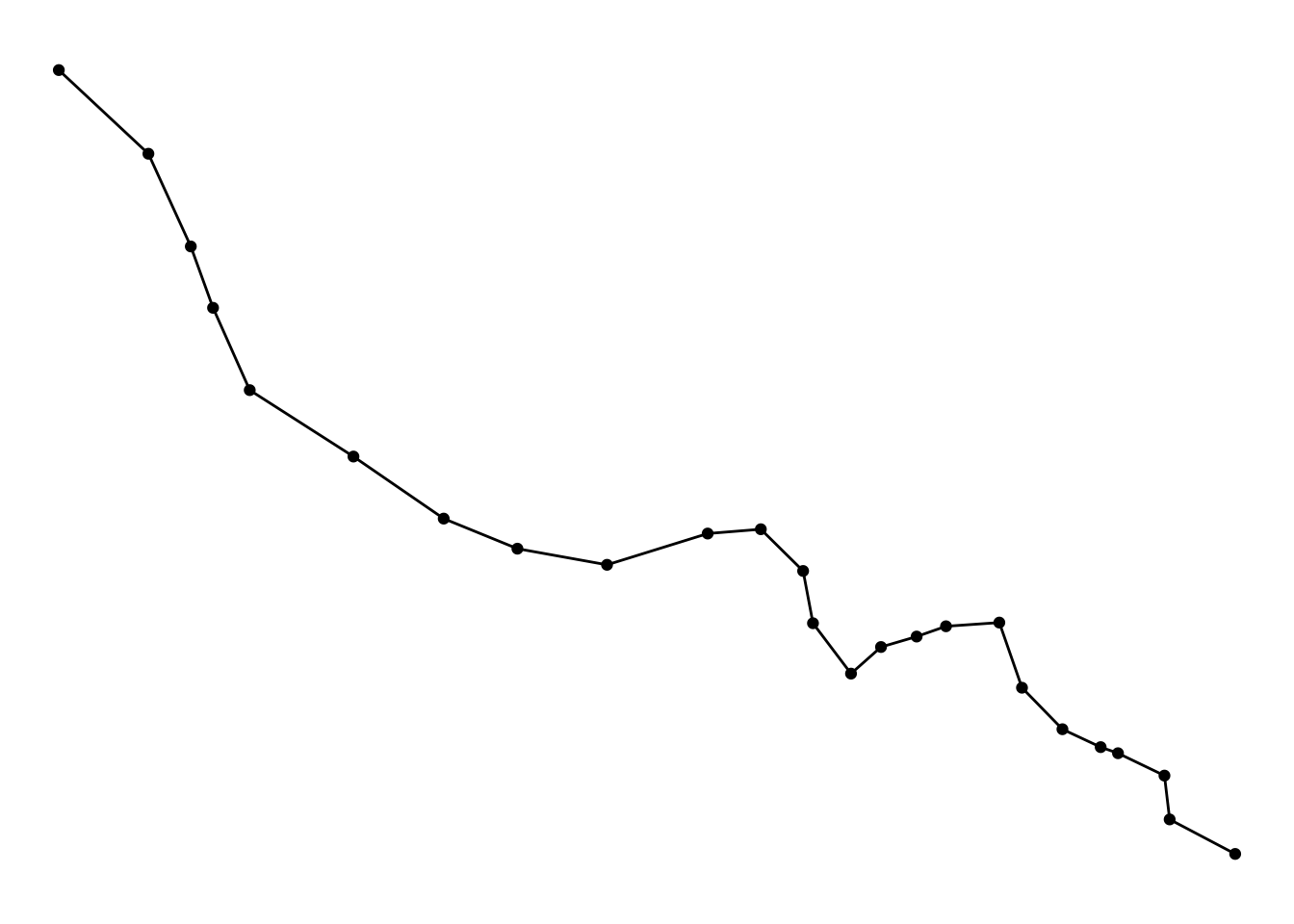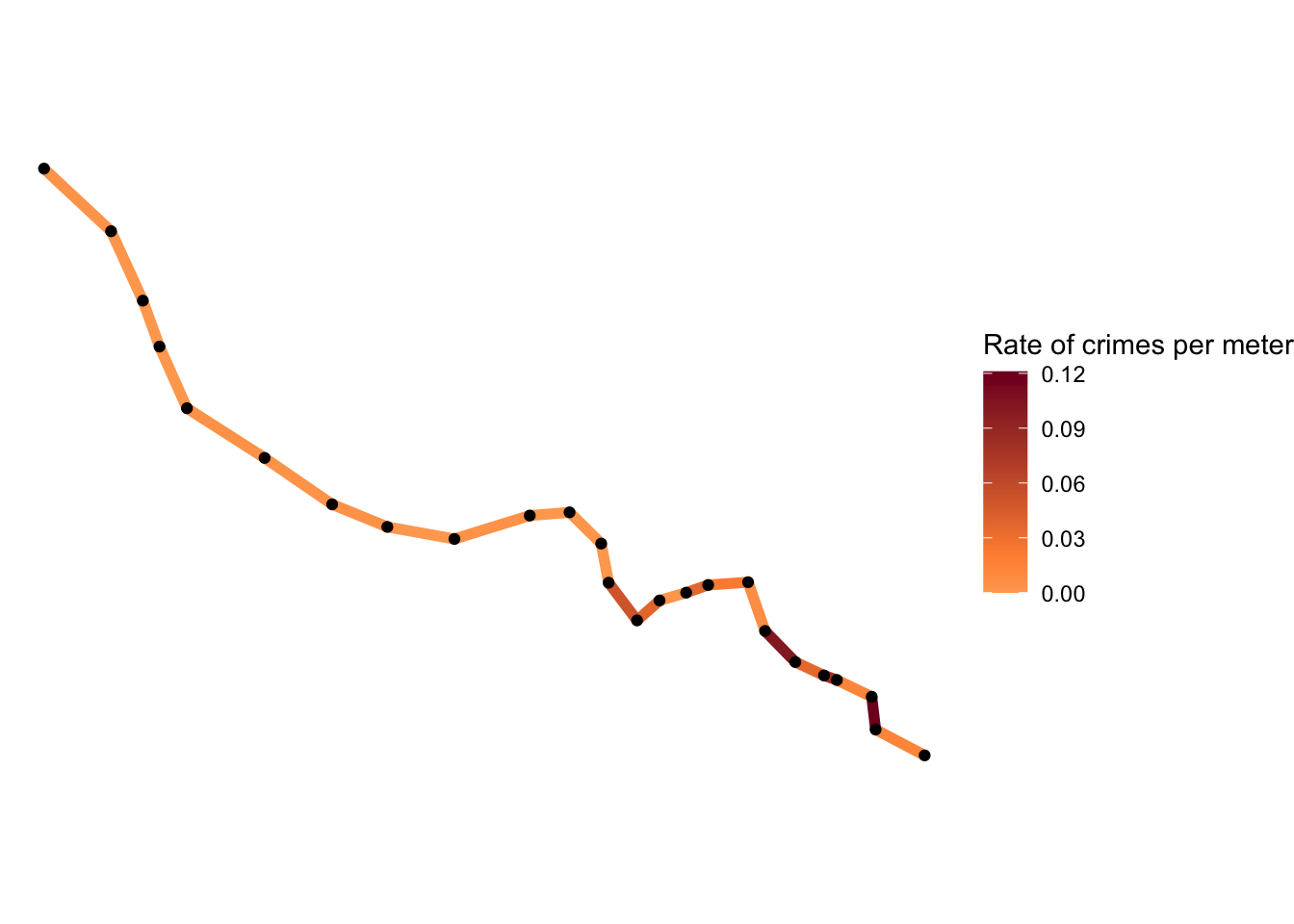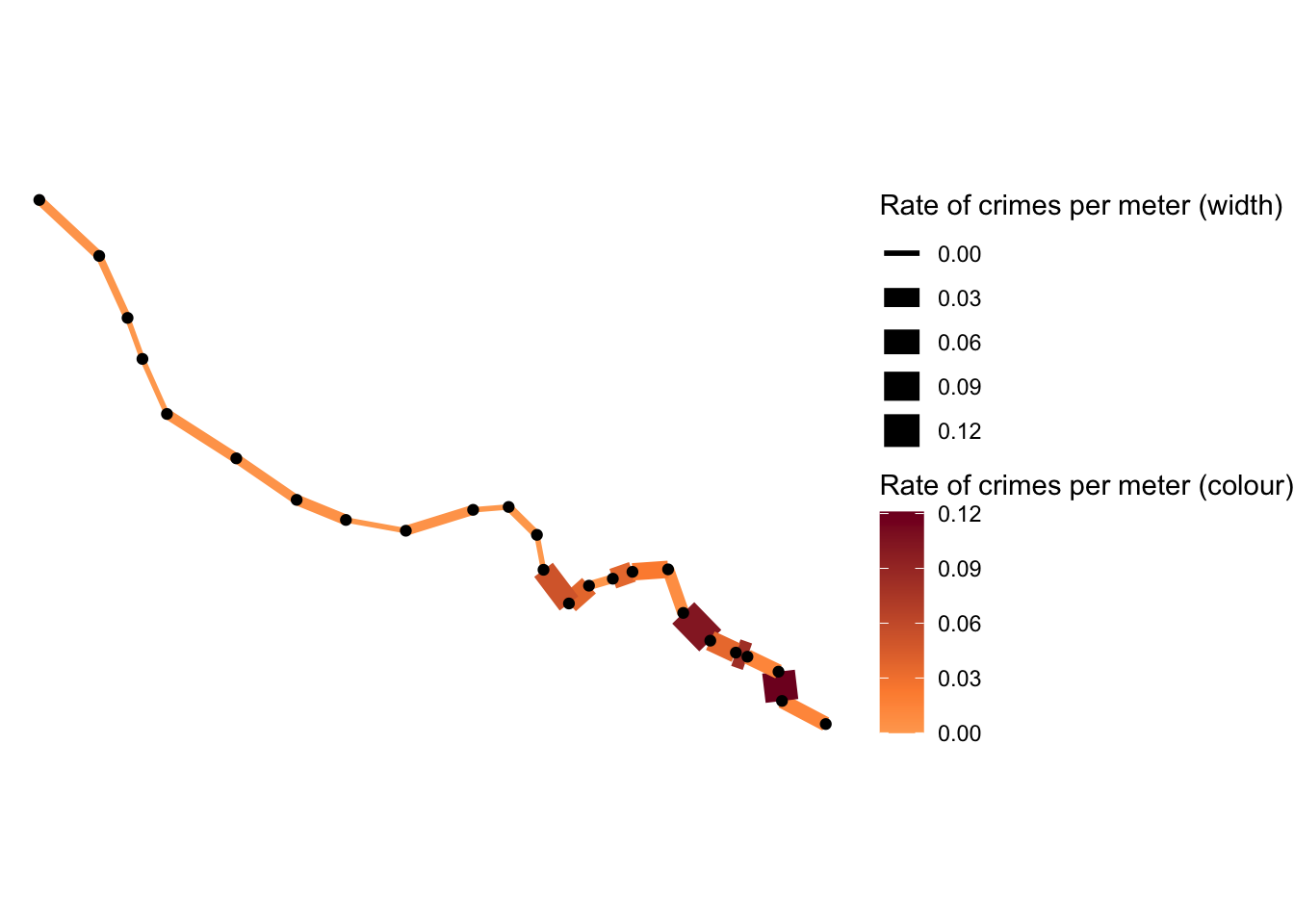# Hot Routes Tutorial## Dr Reka Solymosi

March 29, 2020

Crime concentrates in place and time, and it is the task of the crime analyst to identify where and when these hotspots emerge. This is often achieved by producing density maps. Kernel density estimation is one methdod for producing such maps, which involves applying a function (known as a “kernel”) to each data point, which averages the location of that point with respect to the location of other data points. The surface that results from this model allows us to produce isarithmic maps, also referred to in common parlor as heatmaps (see our crime mapping textbook on GitHub for more on Kernel Density Mapping and a “how-to-in-R” tutorial).

However, often we might be interested in how crime concentrates along a particular network such as along streets, bus routes, train nextworks, and others. In order to map hot spots along a network, we can use a technique called hot routes. It was used as early as 2003 by Andrew Newton to map crime and disorder on the bus network in Merseyside. A useful how-to guide was later produced by Henry Partridge and Lisa Tompson and can be accessed in the associated JDI brief or journal article.

Hot Routes was devised to be a straightforward spatial technique that analyses crime patterns that are associated with a linear network (e.g. streets and other transportation networks). It allows an analyst to map crime concentrations along different segments of the network and visualise this through colour.

Here I will go through an example of how to apply hot routes in R. We will follow the 4 steps outlined by Henry Partridge and Lisa Tompson in the JDI brief:

Step 1: Prepare the network layer Step 2. Link crime events to street segments Step 3: Calculate a rate Step 4: Visualise the results

I will use the example dataset of the London Underground network, spatial data for which can be downloaded using the Transport for London API, and crime from British Transport Police for February 2020 available via data.police.uk.

## Step 1: Prepare the network layer

The first step is to prepare our network layer. There are several substeps to this. First we need to acquire a shapefile for our network. Here we will keep it simple for the sake of the tutorial, and use only one line. Let’s go with the Bakerloo line. We can get this data using the TfL API. We can use R to make the API call for us by taking the query URL, and getting the results using the `readLines()` function in base R, and putting that within the `fromJSON()` function from the`rjson` package.

``````library(rjson)

#get json from TfL API
``````## Warning in readLines("https://api.tfl.gov.uk/line/bakerloo/route/sequence/
## outbound"): incomplete final line found on 'https://api.tfl.gov.uk/line/
## bakerloo/route/sequence/outbound'``````

When you run the above, you might get a warning messae about incomplete final line, I’m not sure why, but we still get the object `api_call` in our environment, you can see it’s a large list with 10 elements.

You can have a look at this list, it has many interesting bits, but what I want to do here is extract the stops along the bakerloo line and their respective coordinates. There are probably much more efficient ways to do this, but here is mine (this is a tutorial on hot routes, not on parsing json files haha!)

``````#parse df of stops and latlongs
datalist = list()
for (i in 1:length(api_call\$stations)) {

datalist[[i]] <- data.frame(stn_name = api_call\$stations[[i]]\$name,
stn_lat = api_call\$stations[[i]]\$lat,
stn_lon = api_call\$stations[[i]]\$lon,
line = "bakerloo")
}
bakerloo_stops <- do.call(rbind, datalist)``````

Now you can see we have an object called `bakerloo_stops` which has 25 observations of 4 variables (station name, latitude, longitude, and line).

In order to carry out our spatial operations, we will be making use of the `sf` package. So I will load the sf package and also convert this list of stops to an sf object using `st_as_sf()` and build the line between the stops using `group_by()` (from dplyr package), `st_union()` and `st_cast()` (from sf):

``````library(dplyr)
library(sf)

bakerloo_stops <- st_as_sf(bakerloo_stops, coords = c("stn_lon", "stn_lat"), crs = 4326)

bakerloo_line <- bakerloo_stops %>%
group_by(line) %>%
st_union() %>%
st_cast("LINESTRING")``````

Now we have a line and the set of stations along it. We can use the `ggplot2` package to plot it like so:

``````library(ggplot2)

ggplot()+
geom_sf(data = bakerloo_stops) +
geom_sf(data = bakerloo_line) +
theme_void() +
theme(panel.grid.major = element_line(colour = "white"))``````NOTE: I have added `theme_void()` AND `theme(panel.grid.major = element_line(colour = "white"))` because apprently theme_void() + geom_sf() have some issues together.

Right, so that’s looking good. But here we have only one line. What we want is to break our line into sections. How you do this depends really much on what you want to show. In this case, it might be meaningful to consider each segment of the line between stops. In this case, we can use the shapefile of the stops (`bakerloo_stops`) to break up our line (`bakerloo_line`). However, as Henry and Lisa note, network layers typically contain streets of unequal length. This means that longer segments might show up as hot simply because they have more space to contain more crimes. Therefore in such cases it is advisable in this analysis to use equal length street segments, where possible. In this case however, let’s stick to the stops. To split our linestring (`bakerloo_line`) into many linestrings using the stops (`bakerloo_stops`) we can use the `st_split()` function from the `lwgeom` package and `st_collection_extract()` function from `sf`. Furter, as the `st_split()` function is expecting a blade argument of length 1, we can use the `st_combine()` (from sf) function to group our tube stations alltogether:

``````library(lwgeom)

parts <- st_split(bakerloo_line, st_combine(bakerloo_stops\$geometry)) %>% st_collection_extract("LINESTRING")

parts``````
``````## Geometry set for 24 features
## Geometry type: LINESTRING
## Dimension:     XY
## Bounding box:  xmin: -0.334896 ymin: 51.4945 xmax: -0.099185 ymax: 51.59222
## Geodetic CRS:  WGS 84
## First 5 geometries:``````

You can see we now have a new object called `parts` which is a linestring containing 24 features, the segments between our 25 tube stations, all as unique lines. You can also see that this is a geometry set of 24 features, let’s turn it into a simple features collection, and label each of the segments with a number by taking each element of parts and binding it together as a dataframe.

``````datalist = list()
for (i in 1:length(parts)) {

datalist[[i]] <- st_as_sf(data.frame(section = i), geometry = st_geometry(parts[i]))

}

bakerloo_sections <- do.call(rbind, datalist)``````

## Step 3: Calculate a rate

Next, to calculate a rate we need a denominator. In this case, length may be a good one, so the length of each street segment needs to be calculated. We can do this using the `st_length()` function. Since our data are all in WGS84 projection, this will return the length of each segment in meters.

``bakerloo_sections\$length <- st_length(bakerloo_sections)``

Once we have all the lengths, a new column needs to be created in the network layer to record a crime per metre score. This is calculated by dividing the number of crimes linked to a street segment by its length.

``bakerloo_sections\$crime_per_m <- bakerloo_sections\$Freq / bakerloo_sections\$length``

We now have our crimes per meter score! On to mapping!

## Step 4: Visualise the results

The final step is to thematically shade each street segment with a colour (and line thickness if desired) that corresponds to the range of the rate of crime per metre.

For this, let’s convert our crimes per meter (`crime_per_m`) variable to numeric from a “units” object, and then use ggplot once again.

``````bakerloo_sections\$crime_per_m <- as.numeric(bakerloo_sections\$crime_per_m)

midpoint_rates <- mean(bakerloo_sections\$crime_per_m)

ggplot() +
geom_sf(data = bakerloo_sections, aes(colour = crime_per_m), lwd = 2) +
geom_sf(data = bakerloo_stops) +
theme_void() +
theme(panel.grid.major = element_line(colour = "white")) +  #theme void is buggy with geom_sf() so need this too
scale_colour_gradient2(name = "Rate of crimes per meter",
midpoint = midpoint_rates,
low = "#ffffcc", mid = "#fd8d3c", high = "#800026")``````And if we wanted to add thickness as well we can by specifying the size argument:

``````ggplot() +
geom_sf(data = bakerloo_sections, aes(colour = crime_per_m, size = crime_per_m), show.legend = "line") +
geom_sf(data = bakerloo_stops) +
theme_void() +
theme(panel.grid.major = element_line(colour = "white")) +  #theme void is buggy with geom_sf() so need this too
scale_colour_gradient2(name = "Rate of crimes per meter (colour)",
midpoint = midpoint_rates,
low = "#ffffcc", mid = "#fd8d3c", high = "#800026") +
scale_size_continuous(name = "Rate of crimes per meter (width)") ``````All done! We have now managed to create a hot routes map of crimes on or near the barkerloo line recorded by British Transport Police in February 2020.

## Final remarks

This has been a very simple application of the hot routes technique in R, and I hope this tutorial is helpful and can be applied to many other sets of data! If anyone is following along and gets stuck just get in touch with me on twitter ([@r_solymosi](https://twitter.com/r_solymosi)) or by email reka.solymosi@manchester.ac.uk. Also many of the solutions here are possibly hacky, if someone has better ways of doing this please just let me know, I am happy to improve it. As is always the case there are more sophisticated ways to do this, for example using the `spatstat` package (for a quick intro see section 6.7 in our crime mapping textbook), and probably many more, but this also does the job…!

Further, while hot routes is a really neat way to visualise crime rates along a network for sure, it may be the case that the geographic location of each segment or stop is not so important, and instead it is the ditribution of crime along a route that is the focus. In this case another visualisation technique that may be useful is street profile analysis introduced by Valerie Spicer and colleagues, detailed in this paper. I can always write another tutorial on this one in R, if there’s interest.

Anyway, happy mapping friends!